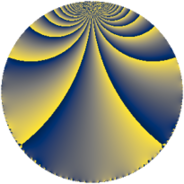# Properties

 Label 2880.2.f.nLevel $2880$ Weight $2$ Character orbit 2880.f Analytic conductor $22.997$ Analytic rank $0$ Dimension $2$ CM discriminant -4 Inner twists $4$

# Learn more about

## Newspace parameters

 Level: $$N$$ $$=$$ $$2880 = 2^{6} \cdot 3^{2} \cdot 5$$ Weight: $$k$$ $$=$$ $$2$$ Character orbit: $$[\chi]$$ $$=$$ 2880.f (of order $$2$$, degree $$1$$, not minimal)

## Newform invariants

 Self dual: no Analytic conductor: $$22.9969157821$$ Analytic rank: $$0$$ Dimension: $$2$$ Coefficient field: $$\Q(\sqrt{-1})$$ Defining polynomial: $$x^{2} + 1$$ Coefficient ring: $$\Z[a_1, \ldots, a_{5}]$$ Coefficient ring index: $$2$$ Twist minimal: no (minimal twist has level 160) Sato-Tate group: $\mathrm{U}(1)[D_{2}]$

## $q$-expansion

Coefficients of the $$q$$-expansion are expressed in terms of $$i = \sqrt{-1}$$. We also show the integral $$q$$-expansion of the trace form.

 $$f(q)$$ $$=$$ $$q + ( 1 - 2 i ) q^{5} +O(q^{10})$$ $$q + ( 1 - 2 i ) q^{5} + 4 i q^{13} -8 i q^{17} + ( -3 - 4 i ) q^{25} -10 q^{29} -12 i q^{37} + 10 q^{41} + 7 q^{49} + 4 i q^{53} -10 q^{61} + ( 8 + 4 i ) q^{65} -16 i q^{73} + ( -16 - 8 i ) q^{85} -10 q^{89} -8 i q^{97} +O(q^{100})$$ $$\operatorname{Tr}(f)(q)$$ $$=$$ $$2q + 2q^{5} + O(q^{10})$$ $$2q + 2q^{5} - 6q^{25} - 20q^{29} + 20q^{41} + 14q^{49} - 20q^{61} + 16q^{65} - 32q^{85} - 20q^{89} + O(q^{100})$$

## Character values

We give the values of $$\chi$$ on generators for $$\left(\mathbb{Z}/2880\mathbb{Z}\right)^\times$$.

 $$n$$ $$577$$ $$641$$ $$901$$ $$2431$$ $$\chi(n)$$ $$-1$$ $$1$$ $$1$$ $$1$$

## Embeddings

For each embedding $$\iota_m$$ of the coefficient field, the values $$\iota_m(a_n)$$ are shown below.

For more information on an embedded modular form you can click on its label.

Label $$\iota_m(\nu)$$ $$a_{2}$$ $$a_{3}$$ $$a_{4}$$ $$a_{5}$$ $$a_{6}$$ $$a_{7}$$ $$a_{8}$$ $$a_{9}$$ $$a_{10}$$
1729.1
 1.00000i − 1.00000i
0 0 0 1.00000 2.00000i 0 0 0 0 0
1729.2 0 0 0 1.00000 + 2.00000i 0 0 0 0 0
 $$n$$: e.g. 2-40 or 990-1000 Significant digits: Format: Complex embeddings Normalized embeddings Satake parameters Satake angles

## Inner twists

Char Parity Ord Mult Type
1.a even 1 1 trivial
4.b odd 2 1 CM by $$\Q(\sqrt{-1})$$
5.b even 2 1 inner
20.d odd 2 1 inner

## Twists

By twisting character orbit
Char Parity Ord Mult Type Twist Min Dim
1.a even 1 1 trivial 2880.2.f.n 2
3.b odd 2 1 320.2.c.a 2
4.b odd 2 1 CM 2880.2.f.n 2
5.b even 2 1 inner 2880.2.f.n 2
8.b even 2 1 1440.2.f.c 2
8.d odd 2 1 1440.2.f.c 2
12.b even 2 1 320.2.c.a 2
15.d odd 2 1 320.2.c.a 2
15.e even 4 1 1600.2.a.l 1
15.e even 4 1 1600.2.a.m 1
20.d odd 2 1 inner 2880.2.f.n 2
24.f even 2 1 160.2.c.a 2
24.h odd 2 1 160.2.c.a 2
40.e odd 2 1 1440.2.f.c 2
40.f even 2 1 1440.2.f.c 2
40.i odd 4 1 7200.2.a.y 1
40.i odd 4 1 7200.2.a.bb 1
40.k even 4 1 7200.2.a.y 1
40.k even 4 1 7200.2.a.bb 1
48.i odd 4 1 1280.2.f.c 2
48.i odd 4 1 1280.2.f.d 2
48.k even 4 1 1280.2.f.c 2
48.k even 4 1 1280.2.f.d 2
60.h even 2 1 320.2.c.a 2
60.l odd 4 1 1600.2.a.l 1
60.l odd 4 1 1600.2.a.m 1
120.i odd 2 1 160.2.c.a 2
120.m even 2 1 160.2.c.a 2
120.q odd 4 1 800.2.a.e 1
120.q odd 4 1 800.2.a.f 1
120.w even 4 1 800.2.a.e 1
120.w even 4 1 800.2.a.f 1
240.t even 4 1 1280.2.f.c 2
240.t even 4 1 1280.2.f.d 2
240.bm odd 4 1 1280.2.f.c 2
240.bm odd 4 1 1280.2.f.d 2

By twisted newform orbit
Twist Min Dim Char Parity Ord Mult Type
160.2.c.a 2 24.f even 2 1
160.2.c.a 2 24.h odd 2 1
160.2.c.a 2 120.i odd 2 1
160.2.c.a 2 120.m even 2 1
320.2.c.a 2 3.b odd 2 1
320.2.c.a 2 12.b even 2 1
320.2.c.a 2 15.d odd 2 1
320.2.c.a 2 60.h even 2 1
800.2.a.e 1 120.q odd 4 1
800.2.a.e 1 120.w even 4 1
800.2.a.f 1 120.q odd 4 1
800.2.a.f 1 120.w even 4 1
1280.2.f.c 2 48.i odd 4 1
1280.2.f.c 2 48.k even 4 1
1280.2.f.c 2 240.t even 4 1
1280.2.f.c 2 240.bm odd 4 1
1280.2.f.d 2 48.i odd 4 1
1280.2.f.d 2 48.k even 4 1
1280.2.f.d 2 240.t even 4 1
1280.2.f.d 2 240.bm odd 4 1
1440.2.f.c 2 8.b even 2 1
1440.2.f.c 2 8.d odd 2 1
1440.2.f.c 2 40.e odd 2 1
1440.2.f.c 2 40.f even 2 1
1600.2.a.l 1 15.e even 4 1
1600.2.a.l 1 60.l odd 4 1
1600.2.a.m 1 15.e even 4 1
1600.2.a.m 1 60.l odd 4 1
2880.2.f.n 2 1.a even 1 1 trivial
2880.2.f.n 2 4.b odd 2 1 CM
2880.2.f.n 2 5.b even 2 1 inner
2880.2.f.n 2 20.d odd 2 1 inner
7200.2.a.y 1 40.i odd 4 1
7200.2.a.y 1 40.k even 4 1
7200.2.a.bb 1 40.i odd 4 1
7200.2.a.bb 1 40.k even 4 1

## Hecke kernels

This newform subspace can be constructed as the intersection of the kernels of the following linear operators acting on $$S_{2}^{\mathrm{new}}(2880, [\chi])$$:

 $$T_{7}$$ $$T_{11}$$ $$T_{13}^{2} + 16$$ $$T_{17}^{2} + 64$$ $$T_{19}$$ $$T_{29} + 10$$

## Hecke characteristic polynomials

$p$ $F_p(T)$
$2$ $$T^{2}$$
$3$ $$T^{2}$$
$5$ $$5 - 2 T + T^{2}$$
$7$ $$T^{2}$$
$11$ $$T^{2}$$
$13$ $$16 + T^{2}$$
$17$ $$64 + T^{2}$$
$19$ $$T^{2}$$
$23$ $$T^{2}$$
$29$ $$( 10 + T )^{2}$$
$31$ $$T^{2}$$
$37$ $$144 + T^{2}$$
$41$ $$( -10 + T )^{2}$$
$43$ $$T^{2}$$
$47$ $$T^{2}$$
$53$ $$16 + T^{2}$$
$59$ $$T^{2}$$
$61$ $$( 10 + T )^{2}$$
$67$ $$T^{2}$$
$71$ $$T^{2}$$
$73$ $$256 + T^{2}$$
$79$ $$T^{2}$$
$83$ $$T^{2}$$
$89$ $$( 10 + T )^{2}$$
$97$ $$64 + T^{2}$$
show more
show less